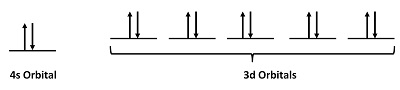# 2.7: Magnetic Properties of Atoms and Ions

Skills to Develop

• To understand the difference between paramagnetism and diamagnetism

The magnetic moment of a system measures the strength and the direction of its magnetism. The term itself usually refers to the magnetic dipole moment. Anything that is magnetic, like a bar magnet or a loop of electric current, has a magnetic moment. A magnetic moment is a vector quantity, with a magnitude and a direction. An electron has an electron magnetic dipole moment, generated by the electron's intrinsic spin property, making it an electric charge in motion. There are many different magnetic forms: including paramagnetism, and diamagnetism, ferromagnetism, and anti-ferromagnetism.

## Paramagnetism

Paramagnetism refers to the magnetic state of an atom with one or more unpaired electrons. The unpaired electrons are attracted by a magnetic field due to the electrons' magnetic dipole moments. Hund's Rule states that electrons must occupy every orbital singly before any orbital is doubly occupied. This may leave the atom with many unpaired electrons. Because unpaired electrons can spin in either direction, they display magnetic moments in any direction. This capability allows paramagnetic atoms to be attracted to magnetic fields. Diatomic oxygen, $$O_2$$ is a good example of paramagnetism (described via molecular orbital theory). The following video shows liquid oxygen attracted into a magnetic field created by a strong magnet:

Figure 2.7.1: As shown in the video, molecular oxygen ($$O_2$$ is paramagnetic and is attracted to the magnet. Incontrast, Molecular nitrogen, $$N_2$$, however, has no unpaired electrons and it is diamagnetic (this concept is discussed below); it is therefore unaffected by the magnet.

There are some exceptions to the paramagnetism rule; these concern some transition metals, in which the unpaired electron is not in a d-orbital. Examples of these metals include $$Sc^{3+}$$, $$Ti^{4+}$$, $$Zn^{2+}$$, and $$Cu^+$$. These metals are the not defined as paramagnetic: they are considered diamagnetic because all d-electrons are paired. Paramagnetic compounds sometimes display bulk magnetic properties due to the clustering of the metal atoms. This phenomenon is known as ferromagnetism, but this property is not discussed here.

## Diamagnetism

Diamagnetic substances are characterized by paired electrons—except in the previously-discussed case of transition metals, there are no unpaired electrons. According to the Pauli Exclusion Principle which states that no two identical electrons may take up the same quantum state at the same time, the electron spins are oriented in opposite directions. This causes the magnetic fields of the electrons to cancel out; thus there is no net magnetic moment, and the atom cannot be attracted into a magnetic field. In fact, diamagnetic substances are weakly repelled by a magnetic field as demonstrated with the pyrolytic carbon sheet in Figure 2.7.2.Figure 2.7.2: Levitating pyrolytic carbon: A small (~6mm) piece of pyrolytic graphite levitating over a permanent neodymium magnet array (5mm cubes on a piece of steel). Note that the poles of the magnets are aligned vertically and alternate (two with north facing up, and two with south facing up, diagonally). Image used with permission from Wikipedia.

## How to tell if a substance is paramagnetic or diamagnetic

The magnetic form of a substance can be determined by examining its electron configuration: if it shows unpaired electrons, then the substance is paramagnetic; if all electrons are paired, the substance is diamagnetic. This process can be broken into four steps:

1. Find the electron configuration
2. Draw the valence orbitals
3. Look for unpaired electrons
4. Determine whether the substance is paramagnetic or diamagnetic

Example 2.7.1: Chlorine Atoms

Step 1: Find the electron configuration

For Cl atoms, the electron configuration is 3s23p5

Step 2: Draw the valence orbitals

Ignore the core electrons and focus on the valence electrons only.Step 3: Look for unpaired electrons

There is one unpaired electron.

Step 4: Determine whether the substance is paramagnetic or diamagnetic

Since there is an unpaired electron, Cl atoms are paramagnetic (albeit, weakly).

Example 2.7.2: Zinc Atoms

Step 1: Find the electron configuration

For Zn atoms, the electron configuration is 4s23d10

Step 2: Draw the valence orbitalsStep 3: Look for unpaired electrons

There are no unpaired electrons.

Step 4: Determine whether the substance is paramagnetic or diamagnetic

Because there are no unpaired electrons, Zn atoms are diamagnetic.

Exercise 2.7.1

1. How many unpaired electrons are found in oxygen atoms ?
2. How many unpaired electrons are found in bromine atoms?
3. Indicate whether boron atoms are paramagnetic or diamagnetic.
4. Indicate whether F- ions are paramagnetic or diamagnetic.
5. Indicate whether Fe2+ ions are paramagnetic or diamagnetic.
The O atom has 2s22p4 as the electron configuration. Therefore, O has 2 unpaired electrons.